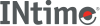﻿ ldivINtime SDK Help
ldiv

Divides numerator by denominator, and computes the quotient and remainder.

```#include <stdlib.h>

ldiv_t ldiv (long int numer, long int denom);
```

#### Parameters

`numer`
Numerator.
`denom`
Denominator. If 0, errno is set to EDOM and 0 returned for quotient and remainder.

#### Remarks

The sign of the quotient is the same as that of the mathematical quotient. Its absolute value is the largest integer that is less than the absolute value of the mathematical quotient.

ldiv is similar to div, except that the arguments and the members of the returned structure are long integers.

#### Return Values

An LDIV_T structure, comprising both the quotient and the remainder, defined in `stdlib.h`.

#### Requirements

Versions Defined in Include Link to
INtime 3.0 intime/rt/include/stdlib.h stdlib.h clib.lib